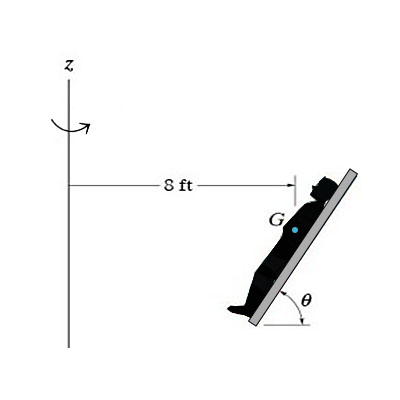# The 165 lb man lies against the cushion for which the coefficient of static friction is \mu_s =...

## Question:

The {eq}165 lb {/eq} man lies against the cushion for which the coefficient of static friction is {eq}\mu_s = 0.5 {/eq}. Due to rotation about the {eq}z {/eq} axis, he has a constant speed {eq}v = 20 ft/s {/eq}. Neglect the size of the man. Take {eq}\theta = 60^o. {/eq}

Part A

Determine the magnitude of the resultant normal force the cushion exerts on him.

Express your answer to three significant figures and include the appropriate units.

Part B

Determine the magnitude of the resultant frictional force the cushion exerts on him.

Express your answer to three significant figures and include the appropriate units## Friction Force

The friction force is the force which opposes the motion of the body, the friction force mainly depends on the type of surface on which object is placed or moving and the normal reaction force applied to the body.

Become a Study.com member to unlock this answer! Create your account

Given Data

• The weight of the man is: {eq}W = 165\;{\rm{lb}} {/eq}
• The coefficient of static friction is: {eq}{\mu _s} = 0.5 {/eq}
• The constant...What is Friction? - Definition, Formula & Forces

from

Chapter 11 / Lesson 22
260K

Friction is the force that opposes the motion of an object. Learn about the sources of friction, identify examples of friction and test your knowledge with quiz questions.